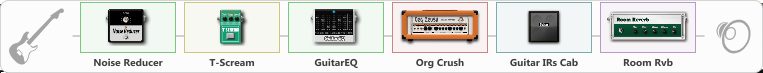# An Go

Discussion in 'ToneLib-GFX presets' started by An go, Mar 24, 2021.

1. An Go

Preset name: Speed

Effects chain:Effect: "Noise Reducer" (Dynamics / Filter), active - "yes"
{
"Sens" = 100
"Mode" = Hard
}

Effect: "T-Scream" (Overdrive / Distortion), active - "yes"
{
"Drive" = 40
"Tone" = 0
"Level" = 79
}

Effect: "GuitarEQ" (Dynamics / Filter), active - "yes"
{
"160 Hz" = -7
"400 Hz" = -9
"800 Hz" = 0
"1.6 kHz" = 0
"3.2 kHz" = 0
"6.4 kHz" = -15
"12 kHz" = 0
"Level (dB)" = 0
}

Effect: "Org Crush" (Amp simulators), active - "yes"
{
"Gain" = 46
"Bass" = 0
"Middle" = 69
"Treble" = 74
"Presence" = 65
"Master" = 61
"Level (dB)" = 0
}

Effect: "Guitar IRs Cab" (Cabinets), active - "yes"
{
"Model" = Marshall JCM2000 (4x12")
"Mic Position" = Middle
"Mic Distance" = Near
"Low Cut (Hz)" = 77
"Hi Cut (kHz)" = 15.5
"Mix" = 100
"Level (dB)" = 0
}

Effect: "Room Rvb" (Reverberation), active - "yes"
{
"Time" = 6.8
"PreDelay" = 0
"LoDamp" = 55
"HiDamp" = 35
"Mix" = 80
}

Note: You will need to download and install the ToneLib-GFX software to use the preset.

File size:
612 bytes
Views:
2,781